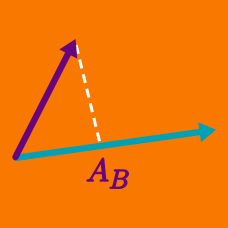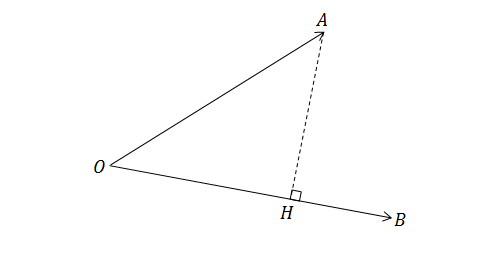Geometry

# Dot Product - Properties

If $\vec{u} = \langle 1, 2, 3 \rangle$ and $\vec{v} = \langle 4, 5, 6\rangle$, then what is $\vec{u} \cdot \vec{v}$?In the figure above, the point $H$ is the perpendicular foot from point $A$ on $\overrightarrow{OB}.$ If $\lvert\overline{OA}\rvert=7,\lvert\overline{OB}\rvert=9,$ and $\lvert\overline{OH}\rvert=5,$ what is $\overrightarrow{OA}\cdot\overrightarrow{OB}?$

Note: The figure is not drawn to scale.

If $\vec{u}, \vec{v},$ and $\vec{w}$ are vectors, then which of the following is not a valid operation?

\begin{aligned} \text{A. } & \hspace{.35cm} (\vec{u}\cdot\vec{v}) + |\vec{w}| \\ \text{B. } & \hspace{.35cm} \vec{u}\cdot\vec{v}\cdot\vec{w} \\ \text{C. } & \hspace{.35cm} \vec{u}\cdot(\vec{v} - \vec{w}) \end{aligned}

Remember! Both inputs for a dot product must be vectors.

If the vectors $\vec{v}=(\sqrt{3},4,\sqrt{2})$ and $\vec{u}=(\sqrt{6},0,k)$ are perpendicular, what must be the value of $k?$

Hint: If two vectors are perpendicular, then their dot product is 0.

If $\vec{a}\cdot\vec{b}=3, \vec{a}\cdot\vec{c}=4, \vec{b}\cdot\vec{c}=-2,$ and $\lvert\vec{b}\rvert=1,$ then evaluate $(\vec{a}-\vec{b})\cdot(2\vec{b}+3\vec{c})$

×